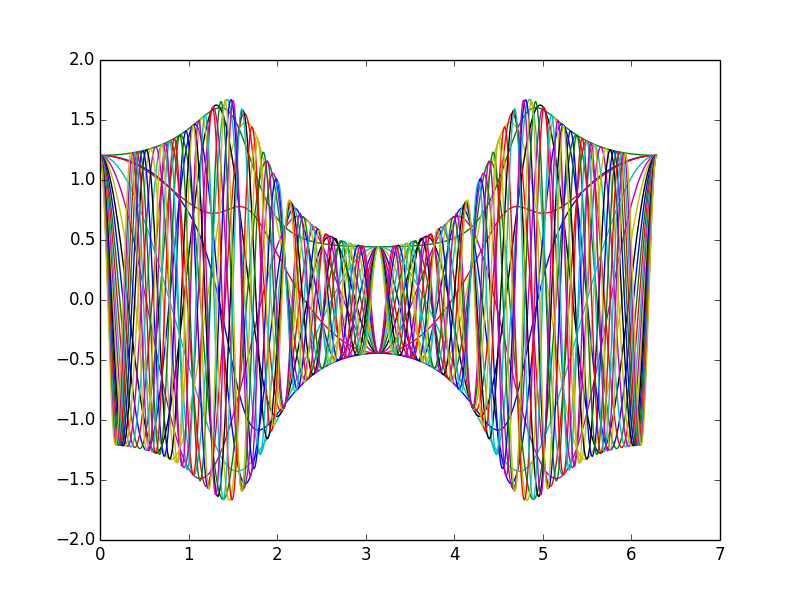# Revision history [back]

A possibility would be to use matplotlib+numpy. For example:

import numpy as np
import matplotlib.pyplot as plt

def get_f( n ):
i=np.complex(0,1)
def func(t):
return np.exp(i*t*(1-n))*(1+(np.exp(np.exp(i*t))-1)/(np.exp(2*np.exp(i*t))+1))
return func

def mat_plot():
t = np.arange(0.,2*np.pi,0.01)
for i in range(20):
func = get_f(i)
ft = func(t)
plt.plot(t,np.real(ft))
plt.show()
plt.clf()


Takes only 0.5s at SMC (and 234ms on my laptop). Sample output: